0/1
##### Introduction
###### Lessons
1. $\cdot$What are "like radicals"?
$\cdot$Only "like radicals" can be combined through addition and subtraction.
0/5
##### Examples
###### Lessons
Combine each expression into a single radical.
1. $\sqrt {90} - \sqrt {10}$
2. ${^3}\sqrt{{40}} + {^3}\sqrt{{135}}$
3. $7\sqrt {72} - 5\sqrt {180} + 10\sqrt {392}$
2. Simplify by combining like radicals.
1. $6\sqrt {24} + \frac{3}{2}\sqrt {20} - \frac{3}{4}\sqrt {96} + \frac{1}{2}\sqrt {180}$
2. $\frac{{{^3}\sqrt{{500}}}}{{10}} + 4{^3}\sqrt{{120}} - \frac{{2{^3}\sqrt{{108}}}}{3} - \frac{{{^3}\sqrt{{405}}}}{2}$
###### Free to Join!
StudyPug is a learning help platform covering math and science from grade 4 all the way to second year university. Our video tutorials, unlimited practice problems, and step-by-step explanations provide you or your child with all the help you need to master concepts. On top of that, it's fun - with achievements, customizable avatars, and awards to keep you motivated.
• #### Easily See Your ProgressWe track the progress you've made on a topic so you know what you've done. From the course view you can easily see what topics have what and the progress you've made on them. Fill the rings to completely master that section or mouse over the icon to see more details.
• #### Make Use of Our Learning Aids###### Practice Accuracy

See how well your practice sessions are going over time.

Stay on track with our daily recommendations.

• #### Earn Achievements as You LearnMake the most of your time as you use StudyPug to help you achieve your goals. Earn fun little badges the more you watch, practice, and use our service.
• #### Create and Customize Your AvatarPlay with our fun little avatar builder to create and customize your own avatar on StudyPug. Choose your face, eye colour, hair colour and style, and background. Unlock more options the more you use StudyPug.
###### Topic Notes

Radicals can be simplified through adding and subtracting, but you should keep in mind that you sometimes can't "cleanly" simplify square roots down into a number. The first thing to note is that radicals can only be added and subtracted if they have the same root number. If the radicals in a question are unlike, you won't be able to combine them together. That's one of the main rules for radicals that you should remember.

Our example will help you grasp the basics of what we mean when we say you have to find like radicals before combining them. The simplifying radicals problem below deals with how to subtract radicals.

Question 1:

$\sqrt{90}-\sqrt{10}$

Solution:

We are going to first simplify this expression. We will perform a division analysis for the number 90 in order to identify its roots:

We learn that $\sqrt{90}$ can be re-written as:

$\sqrt{5 \cdot 2 \cdot 3 \cdot 3}$

Since we are dealing with square roots (which is what a radical sign stands for. There should be a tiny 2 next to the radical sign to indicate that we're working with square roots, but it is generally accepted to simply write the sign without it), we are looking for numbers in pair. In this case, we can see that we have a pair of 3s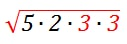Now, we can take the pair outside of the radical (square root), leaving us with:

$3 \sqrt{5 \cdot 2} = 3 \sqrt{10}$

This means our question can be transformed into the following expression:

$3 \sqrt{10} - \sqrt{10}$

You can see that we've now identified the "like" radicals in the question: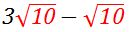.

We can finally do the subtraction, and the result gives us:

$2 \sqrt{10}$

As you can see, the number that we ultimately get isn't a clean, whole number, but rather a number followed by a radical. It's best to leave the number like this because if we take the square root of the radical, we'll get a messy decimal number. Unless if the question tells you to, there's no need to simplify it further. You've already got the simplest radical form via this answer.

In this example, let's take a look to see how to add radicals, as well as deal with a cubic root rather than a square root.

Question 2:

Combine the expression into a single radical.

${^3}\sqrt{40}+{^3}\sqrt{135}$

Solution:

Again, let us first perform a division analysis for ${^3}\sqrt{40}$ and ${^3}\sqrt{135}$

${^3}\sqrt{40}$: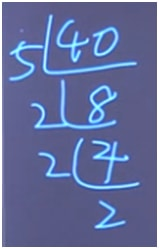the result of division analysis for 40

${^3}\sqrt{135}$: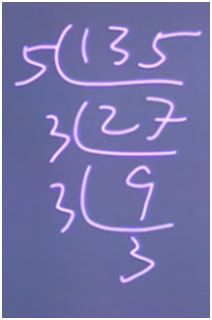the result of division analysis for 135

This allows us to rewrite the original question into this expression:

${^3}\sqrt{5 \cdot 2 \cdot 2 \cdot 2} + {^3}\sqrt{5 \cdot 3 \cdot 3 \cdot 3}$

Since we are dealing with cube root, we are looking for numbers in triplets. In this case, we have a triplet of 2s in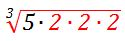and a triplet of 3s in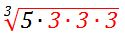Now, we can take the triplets outside of the radical (cubic root), and get this expression:

Now that the two numbers have the same radical: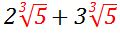We can do the addition, and the result is:
$5{^3}\sqrt{5}$